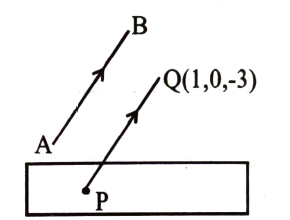Planes in 3D
Question

# Find the distance of the point (1, 0, -3) from the plane x - y -z = 9 measured parallel to the line $\frac{\mathrm{x}-2}{2}=\frac{\mathrm{y}+2}{3}=\frac{\mathrm{z}-6}{-6}$

Moderate
Solution

## Given plane is x - y - z = 9---------(1)Given line AB is $\frac{\mathrm{x}-2}{2}=\frac{\mathrm{y}+2}{3}=\frac{\mathrm{z}-6}{-6}---\left(2\right)$Equation of a line passing through the point Q (1, 0, -3) and parallel to line (2) is $\frac{\mathrm{x}-1}{2}=\frac{\mathrm{y}}{3}=\frac{\mathrm{z}+3}{-6}=\mathrm{r}-----\left(3\right)$Co-ordinates of point on line (3) may be taken as P (2r + 1, 3r, -6r -3)If P is the point of intersection of line (3) and plane (1), then P lies on plane (1),(2r +1)- (3r) - (- 6r-3) = 9 gives r = 1or  $\mathrm{P}\equiv \left(3,3,-9\right)$Distance between points Q(1, 0, -3) and P (3, 3, - 9)$\mathrm{PQ}=\sqrt{\left(3-1{\right)}^{2}+\left(3-0{\right)}^{2}+\left(-9-\left(-3\right){\right)}^{2}}=\sqrt{4+9+36}=7$

Get Instant Solutions